Choose Language Hide Translation Bar
Highlighted

## How to directly calculate the number of consecutive conditions?

For example, take "height" as an example and count the consecutive number of "height"s in the row greater than the "height" in the previous row.

I added an auxiliary column h to determine whether the "height" of the row is greater than the "height" of the previous row, and then counted the consecutive "height" of the row greater than the "height" of the previous row in the other column.If the "height" is less than the "height" of the previous row  have to recalculate.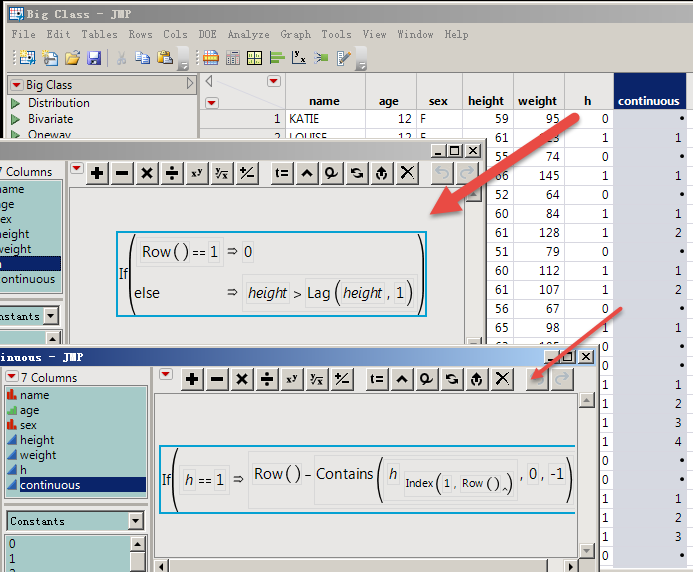• How can do this calculation in one step?

• I tried this, but it didn't work:

• ``if(:height>Lag(:height,1),row()-Contains(:height[Index(1,Row())],:height<Lag(:height,1),-1))``
• Thanks!
12 REPLIES 12
Highlighted

## Re: How to directly calculate the number of consecutive conditions?

The problem has a fairly simple solution:

``````If( Row() == 1,
hCounter = 0,
If( :height > Lag( :height, 1 ),
hCounter++,
hCounter = 0
)
);
If( hCounter == 0,
.,
hCounter
);``````

This version will produce missing values when there are 0 lags that meet the condition, just like your 2 column solution.  If you want the resulting value to be a zero and not a missing value, just use:

``````If( Row() == 1,
hCounter = 0,
If( :height > Lag( :height, 1 ),
hCounter++,
hCounter = 0
)
);

hCounter;``````

Here is a complete comparison of the 2 methods

``````Names Default To Here( 1 );
dt = Open( "\$SAMPLE_DATA/Big Class.jmp" );

dt << New Column( "h",
Numeric,
"Continuous",
Format( "Best", 12 ),
Formula( If( Row() == 1, 0, :height > Lag( :height, 1 ) ) )
);

dt << New Column( "Two Columns Used",
Numeric,
"Continuous",
Format( "Best", 12 ),
Formula( If( :h == 1, Row() - Contains( :h[Index( 1, Row() )], 0, -1 ) ) )
);
dt << New Column( "All in one",
Numeric,
"Continuous",
Format( "Best", 12 ),
Formula(
If( Row() == 1,
hCounter = 0,
If( :height > Lag( :height, 1 ),
hCounter++,
hCounter = 0
)
);
If( hCounter == 0,
.,
hCounter
);
)
);``````
Jim
Highlighted

## Re: How to directly calculate the number of consecutive conditions?

Looping is a good idea here!

Thank Jim!
Highlighted

## Re: How to directly calculate the number of consecutive conditions?

Hi,

I have a similar challenge.  However, I need to calculate the number of sequential (incremented by 1) values in a column.  I attach the BigClass example to illustrate.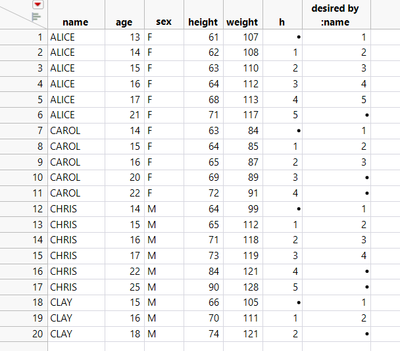I used your formula to calculate column :h based on :age, but desire a formula that will start at the 1st value of a sequence and grouped by :name.

Can this be achieved with a formula?

Regards,

Onjai

Highlighted

## Re: How to directly calculate the number of consecutive conditions?

Just add an IF() statement in the formula that checks to see if the Lag(:Name) != :Name, and if not, then set your counter back to the starting point.

Jim
Highlighted

## Re: How to directly calculate the number of consecutive conditions?

Hi ,

Not quite sure what I am doing wrong, but he If statement is trowing this error.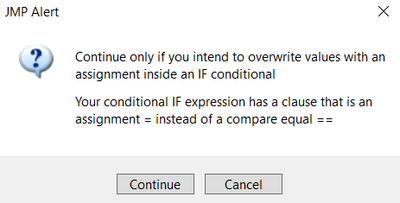for this:

``````If( Row() == 1,
hCounter = 0,
If(
Lag( :name ) = :name,
If( :age > Lag( :age, 1 ),
Empty()
),
hCounter++, hCounter = 0
)
);
If( hCounter == 0,
.,
hCounter
);``````

Highlighted

## Re: How to directly calculate the number of consecutive conditions?

Hi,

Found the error, but the formula still does not calculate column 10 as desired.

Alice's height was measured for 5 consecutive years.  I need a column to show which rows are consecutive.  Clay only had 2 consecutive years of height measurement.  Carol had 3 consecutive years of height measurements but Column 10 shows 4.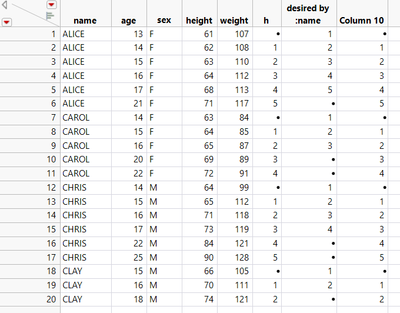Formula in Column 10:

``````If( Row() == 1,
hCounter = 0,
If( Lag( :name ) == :name,
If( :age > Lag( :age, 1 ),
hCounter
++),
hCounter = 0
)
);
If( hCounter == 0,
.,
hCounter
);``````

Highlighted

## Re: How to directly calculate the number of consecutive conditions?

I don't understand the rule for the column "desired by :name".  You have one missing value at the end of the rows for Alice, but 2 rows with missing values for Carol and Chris, and then back to only 1 row of missing values for Clay.  I do not understand

Jim
Highlighted

## Re: How to directly calculate the number of consecutive conditions?

Hi Jim,

No problem.  I named the column to show what I was looking for as an end result of the formula.

Looking at the "desired by:name" column for Carol.

Height measurements were taken for 3 consecutive years (ages 14, 15, 16).  But the formula shows 4.

The difference in age, row to row, should be 1.  If it is anything <>1 then it should be empty.

I need the Lag to increment by 1 until the value is <>1.  Which should signal if a year in height measurement was missed.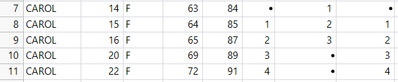Highlighted

## Re: How to directly calculate the number of consecutive conditions?

Hi,

I am attaching a portion of my actual data set.  I am tracking the number of :rolls that are sequential by :master.  The formula is close, but I still need it to set the value of the first occurrence of the sequential roll if the difference is 1.

If( :master == Lag( :master ),
If( Dif( :roll, 1 ) == 1,
Dif( :roll, 1 ),
.
),
.
)

For example, I need to set the value of :sequential roll to 1 for row 8 & 11  because the difference = 1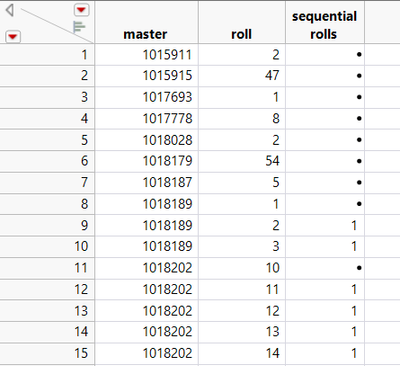Any help would be appreciated.

Article Labels

There are no labels assigned to this post.